Courses

# Power And Index MCQ

## 20 Questions MCQ Test Quantitative Techniques for CLAT | Power And Index MCQ

Description
This mock test of Power And Index MCQ for Quant helps you for every Quant entrance exam. This contains 20 Multiple Choice Questions for Quant Power And Index MCQ (mcq) to study with solutions a complete question bank. The solved questions answers in this Power And Index MCQ quiz give you a good mix of easy questions and tough questions. Quant students definitely take this Power And Index MCQ exercise for a better result in the exam. You can find other Power And Index MCQ extra questions, long questions & short questions for Quant on EduRev as well by searching above.
QUESTION: 1

Solution:
QUESTION: 2

Solution:
QUESTION: 3

### Which of the following is not equal to -27/64

Solution:
QUESTION: 4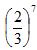is equal to

Solution:
QUESTION: 5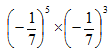is equal to

Solution:
QUESTION: 6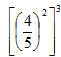is equal to

Solution:
QUESTION: 7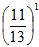is equal to

Solution:
QUESTION: 8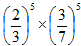is equal to

Solution:
QUESTION: 9

p8 ÷ p2 is equal to (p < o)

Solution:
QUESTION: 10

For any rational numbers p and q , P4 x q4 is equal

Solution:
QUESTION: 11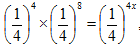the value of x is

Solution:
QUESTION: 12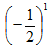is equal to

Solution:
QUESTION: 13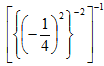is equal to

Solution:
QUESTION: 14

Which of the following is not equal to 1/2?

Solution:
QUESTION: 15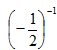is equal to

Solution:
QUESTION: 16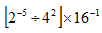is equal to

Solution:
QUESTION: 17

The value of (3-1+4-1)-1 ÷ 5-1 is

Solution:
QUESTION: 18

In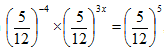the value of x is

Solution:
QUESTION: 19

If (23x-1 + 10) ÷ 7 = 6, the value of x is

Solution: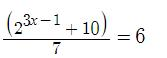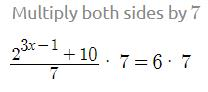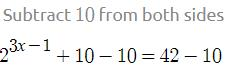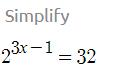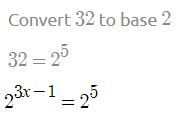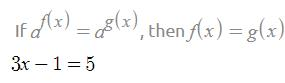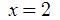QUESTION: 20

The value of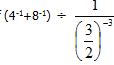is

Solution: